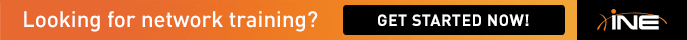# Filtering using ACL

Guys,

Is it possible to match these prefixes using a single permit statement in an access-list?

192.168.5.0/24, .192.168.7.0/24, 192.168.13.0/24, 192.168.15.0/24.

I did it with two.

ip access-l st ROUTES

per 192.168.5.0 0.0.2.255

per 192.168.13.0 0.0.2.255

Thanks

Jeremie

## Comments

• Yes, it is possible.

The third octet is the interesting one. Let's put it in binary:

5 -   00000101

7 -   00000111

13 - 00001101

15 - 00001111

So the pattern we are looking for is 0000x1x1

0000x1x1 - If we fill in the values, the first one is:

00000101 which is 5

then:

00000111 which is 7

then:

00001101 which is 13

then:

00001111 which is 15

So we should ignore bit 2 and bit 4 and check all the rest. These have a value of 2 and 8. 2+8 = 10.

The ACL is then:

192.168.5.0 0.0.10.255

• I was right with you till you said

"So we should ignore bit 2 and bit 4 and check all the rest. These have a value of 2 and 8. 2+8 = 10"

Can you explain "These have a value of 2 and 8"  a bit further?

• It's the bit values

128 64 32 16 8 4 2 1

The second one and the fourth one are the ones we can't check because we had variying numbers in those bit fields.  So the wild card is 00001010 which is 10 in decimal.

• Ahah!!

Well that was a light bulb moment. Use the same thing all the time for summarization, but never quite got the exact science in regards to the wildcard mask. Thanks Daniel.

• Ahah!!

Well that was a light bulb moment. Use the same thing all the time for summarization, but never quite got the exact science in regards to the wildcard mask. Thanks Daniel.

Basically, you could run simple XOR operation in order to get the atcual prefix length for the ACL. Just convert it into the binary as Daniel explained & run the operation, you will get the result as required.

Good luck!

Sign In or Register to comment.